## Unpivot In Google Sheets With Formulas (How To Turn Wide Data Into Tall Data)

Unpivot in Google Sheets is a method to turn “wide” tables into “tall” tables, which are more convenient for analysis.

Suppose we have a wide table like this: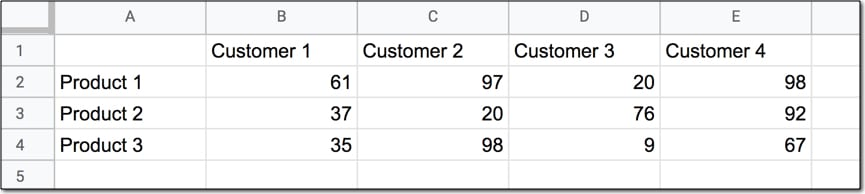Wide data like this is good for the Google Sheets chart tool but it’s not ideal for creating pivot tables or doing analysis. The main reason is that data is captured in the column headings, which prevents you using it in pivot tables for analyis.

So we want to transform this data — unpivot it — into the tall format that is the way databases store data: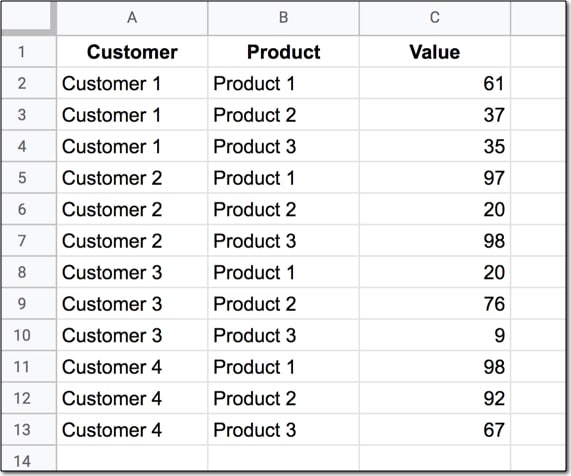But how do we unpivot our data like that?

It turns out it’s quite hard.

It’s harder than going the other direction, turning tall data into wide data tables, which we can do with a pivot table.

This article looks at how to do it using formulas so if you’re ready for some complex formulas, let’s dive in…

We’ll use the wide dataset shown in the first image at the top of this post.

The output of our formulas should look like the second image in this post.

In other words, we need to create 16 rows to account for the different pairings of Customer and Product, e.g. Customer 1 + Product 1, Customer 1 + Product 2, etc. all the way up to Customer 4 + Product 4.

Of course, we’ll employ the Onion Method to understand these formulas.

### Template

Feel free to make your own copy (File > Make a copy…).

(If you can’t open the file, it’s likely because your G Suite account prohibits opening files from external sources. Talk to your G Suite administrator or try opening the file in an incognito browser.)

### Step 1: Combine The Data

Use an array formula like this to combine the column headings (Customer 1, Customer 2, etc.) with the row headings (Product 1, Product 2, Product 3, etc.) and the data.

It’s crucial to add a special character between these sections of the dataset though, so we can split them up later on. I’ve used the fox emoji (because, why not?) but you can use whatever you like, provided it’s unique and doesn’t occur anywhere in the dataset.

`=ArrayFormula(B1:E1&"🦊"&A2:A4&"🦊"&B2:E4)`

The output of this formula is: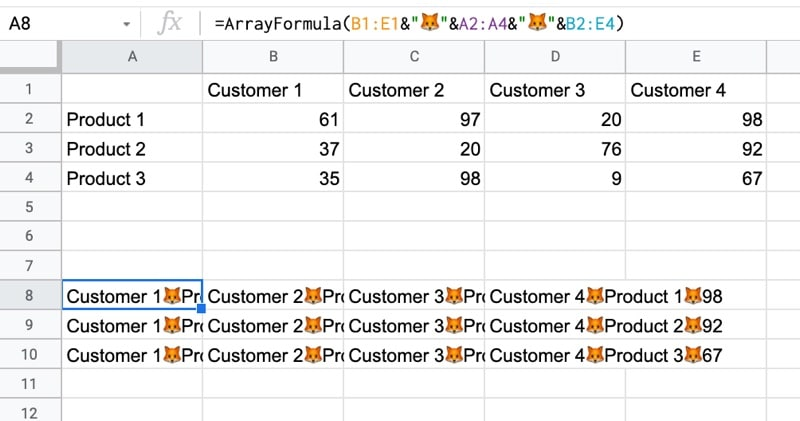### Step 2: Flatten The Data

Before the introduction of the FLATTEN function, this step was much, much harder, involving lots of weird formulas.

Thankfully the FLATTEN function does away with all of that and simply stacks all of the columns in the range on top of each other. So in this example, our combined data turns into a single column.

`=ArrayFormula(FLATTEN(B1:E1&"🦊"&A2:A4&"🦊"&B2:E4))`

The result is: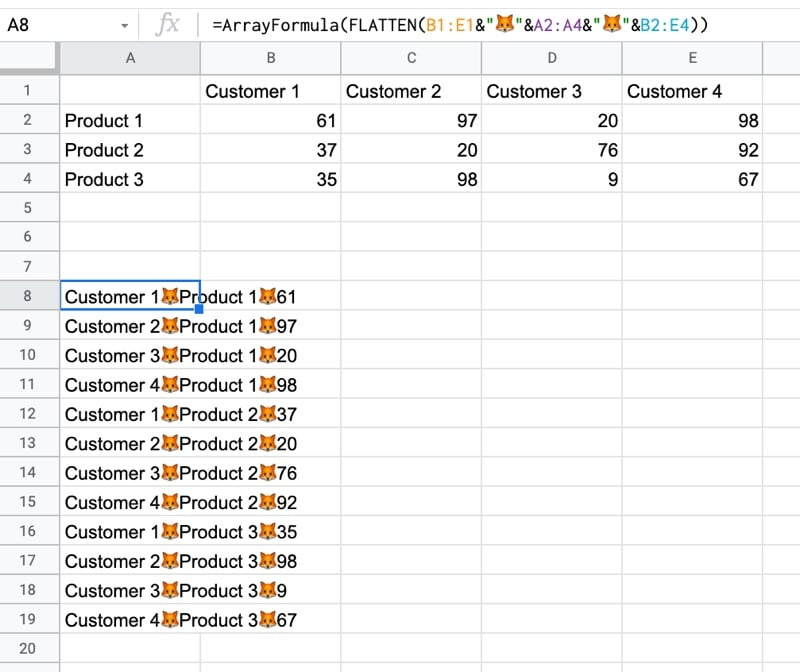### Step 3: Split The Data Into Columns

The final step is to split this new tall column into separate columns for each data type. You can see now why we needed to include the fox emoji so that we have a unique character to split the data on.

Wrap the formula from step 2 with the SPLIT function and set the delimiter to “🦊”:

`=ArrayFormula(SPLIT(FLATTEN(B1:E1&"🦊"&A2:A4&"🦊"&B2:E4),"🦊"))`

This splits the data into the tall data format we want. All that’s left is to add the correct column headings.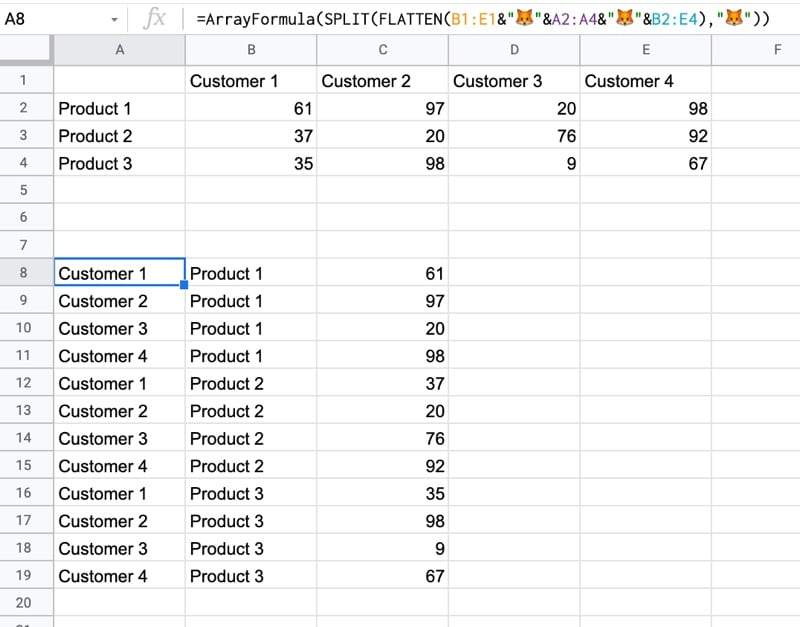## Unpivot With Apps Script

You can also use Apps Script to unpivot data.

Have a look at the example sheet from the first answer of this Stack Overflow post.

## Filtering With Dates In The QUERY Function

If you’ve ever tried to filter on a date column in the Query function in Google Sheets, then you know how tricky it can be.

In a nutshell, the problem occurs because dates in Google Sheets are actually stored as serial numbers, but the Query function requires a date as a string literal in the format yyyy-mm-dd, otherwise it can’t perform the comparison filter.

This post explores this issue in more detail and shows you how to filter with dates correctly in your Query formulas.

## The problem

You might for example try the following syntax:

`=QUERY(Data!\$A\$1:\$H\$136,"select C, B where B > '1/1/2000'",1)`

Unfortunately, the output of such a query is blank: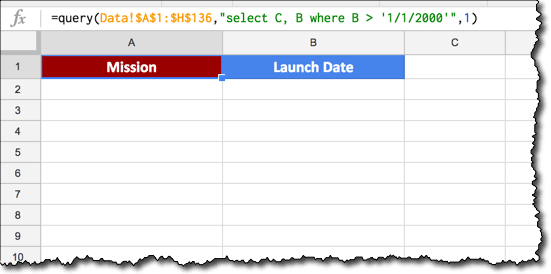If instead we remove the single quotes from around the date and try again, we get a #VALUE! error because the Query formula can’t perform the comparison: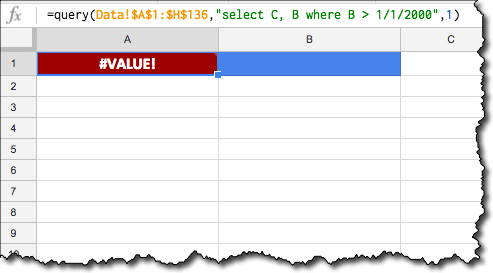Alas, what are we to do!

Neither of these “standard” formats work, because the dates are not in the correct format for the Query function.

## Correct syntax for dates in the Query function

Per the Query Language documentation, we need to include the `date` keyword and ensure that the date is in the format `yyyy-mm-dd` to use a date as a filter in the WHERE clause of our Query function.

Putting aside the Query function for a moment, let’s consider that `"select..."` string.

The new syntax we want will look like this:

`date_column > date '2000-01-01'`

Our challenge is to create a text formula to create this syntax for us, inside our query function.

Dealing with the text function first, starting with our required date of 1/1/2000 and working outwards:

First, we convert it to a serial number format with the `DATEVALUE()` wrapper:

`=DATEVALUE("1/1/2000")`

The output of this formula is a number:

`36526`

Then the `TEXT()` function converts it to the required format for the Query formula by specifying a format of `"yyyy-mm-dd"`:

`=TEXT(DATEVALUE("1/1/2000"),"yyyy-mm-dd")`

The output of this formula is a date in the desired format:

`2000-01-01`

Next we add single quotes around the new date format, with the `"'"` syntax. Finally, we insert the word `date` into the query string, to give:

`="select C, B where B > date '"&TEXT(DATEVALUE("1/1/2000"),"yyyy-mm-dd")&"'"`

which gives or desired output:

`select C, B where B > date '2000-01-01'`

That’s the syntax challenge done!

We can now plop that string into the middle argument of our Query function as per usual, and it’ll do the trick for us.

In this case, I was using a table of Space Shuttle mission data from Wikipedia, which contains a column of launch dates.

I used the `IMPORTHTML()` function to import that table into my Google Sheet, into a tab called Data in the range `A1:H136`. There’s a link to this dataset and worksheet at the end of the post.

This Query formula returns all of the Space Shuttle missions after 1 January 2000:

`=QUERY(Data!\$A\$1:\$H\$136,"select C, B where B > date '"&TEXT(DATEVALUE("1/1/2000"),"yyyy-mm-dd")&"'",1)`

The output of our formula is now returning the correct, filtered data: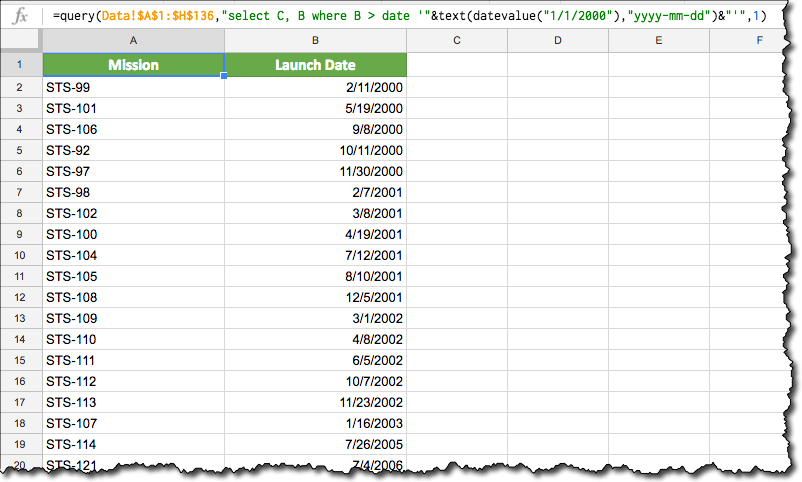## Referencing a date in a cell

The formula is actually simpler in this case, as we don’t need the DATEVALUE function. Assuming we have a date in cell A1 that we want to use in our filter, then the formula becomes:

`=QUERY(Data!\$A\$1:\$H\$136,"select C, B where B > date '"&TEXT(A1,"yyyy-mm-dd")&"'",1)`

## Example showing filter between two dates

Again, it’s relatively simple to extend our formula by adding a second date clause after the `AND` keyword:

`=QUERY(Data!\$A\$1:\$H\$136,"select C, B where B > date '"&TEXT(A1,"yyyy-mm-dd")&"' and B <= date '"&TEXT(B1,"yyyy-mm-dd")&"'",1)`

## Using today’s date as a filter

Substitute the TODAY() function into our formula:

`=QUERY(Data!\$A\$1:\$H\$136,"select C, B where B > date '"&TEXT(TODAY(),"yyyy-mm-dd")&"'",1)`

## How to add a total row to a Query Function table in Google Sheets

This article looks at how to add a total row to tables generated using the Query function in Google Sheets. It’s an interesting use case for array formulas, using the `{...}` notation, rather than the `ArrayFormula` notation.

So what the heck does this all mean?

It means we’re going to see how to add a total row like this:

using an array formula of this form:

`= { QUERY ; { "TOTAL" , SUM(range) } }`

Now of course, at this stage you should be asking:

“But Ben, why not just write the word `TOTAL` under the first column, and `=SUM(range)` in the second column and be done with it?”

Well, it’s a good question so let’s answer it!

The reason for using this method is because the total line is added dynamically, so it will be appended directly at the end of the table, and won’t break if the table expands or contracts, if more data is added.

It’ll always move up or down, so it sits there as the final row.